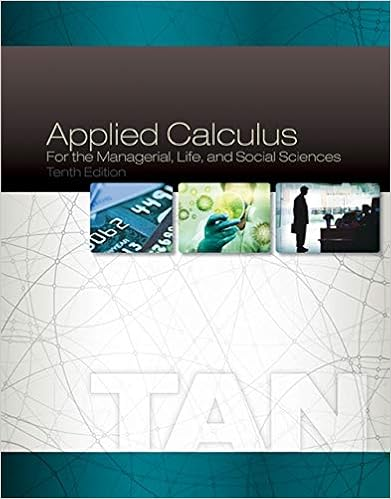Math 211 Practice Final.pdf - MATH 211 NAME PRACTICE FINAL...

• Test Prep
• 12

This preview shows page 1 - 2 out of 12 pages.

We have textbook solutions for you!
The document you are viewing contains questions related to this textbook.The document you are viewing contains questions related to this textbook.
Chapter 2 / Exercise 1
Applied Calculus for the Managerial, Life, and Social Sciences
TanExpert Verified
MATH 211 NAME: PRACTICE FINAL, SPRING 2018 Please show full work on every question. Justify all answers. NO graphing calculators. Anyone caught using a graphing calculator will receive an immediate zero; do not pass GO; do not collect \$200. A scientific calculator is acceptable. This practice exam has been designed to comprehensively cover the material of the semester, and so it is longer than the actual test will be. Any questions that appear on here are fair game for the final. This is not a guarantee that everything on the final is mentioned here. The final exam will be two hours long. 1. Find the derivative of the function f ( x ) = x 3 using the limit definition of the derivative. No credit will be given for an answer that does not use the limit definition of the derivative. (5 min.) 2. Consider the function f ( x ) = e x e x - 1 (1) Find the domain and range of f . Find all asymptotes.
We have textbook solutions for you!
The document you are viewing contains questions related to this textbook.The document you are viewing contains questions related to this textbook.
Chapter 2 / Exercise 1
Applied Calculus for the Managerial, Life, and Social Sciences
TanExpert Verified
•••